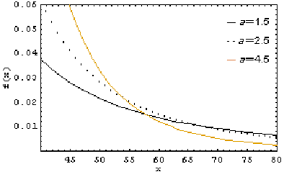## How to Calculate and Solve for P(A) | ProbabilityThe image above represents P(A).

To compute for P(A), two essential parameters are needed and these parameters are Number of Times in Which Event A Can Occur (x) and Total Number of All Possible Outcomes (N).

The formula for calculating P(A):

P(A) = x N

Where;

x = Number of Times in Which Event A can Occur
N = Total Number of All Possible Outcomes

Let’s solve an example;
Find the P(A) when the number of times in which event A can occur is 10 and the total number of all possible outcomes is 22.

This implies that;

x = Number of Times in Which Event A can Occur = 10
N = Total Number of All Possible Outcomes = 22

P(A) = x N
P(A) = 10 22
Dividing the numerator and denominator by 2
P(A) = 5 11
P(A) = 0.45

Therefore, the P(A) is 0.45.